# NPComplete Problems Problems Q Abstract Problems f Decision

• Slides: 16NP-Complete Problems • Problems Q Abstract Problems f Decision Problem, Optimal value, Optimal solution Q Encodings f //Data Structure Q Concrete Problem f //Language • Class of Problems Q P Q NP-Complete f NP-Completeness Proofs • Solving hard problems Q Approximation Algorithms TECH Computer Science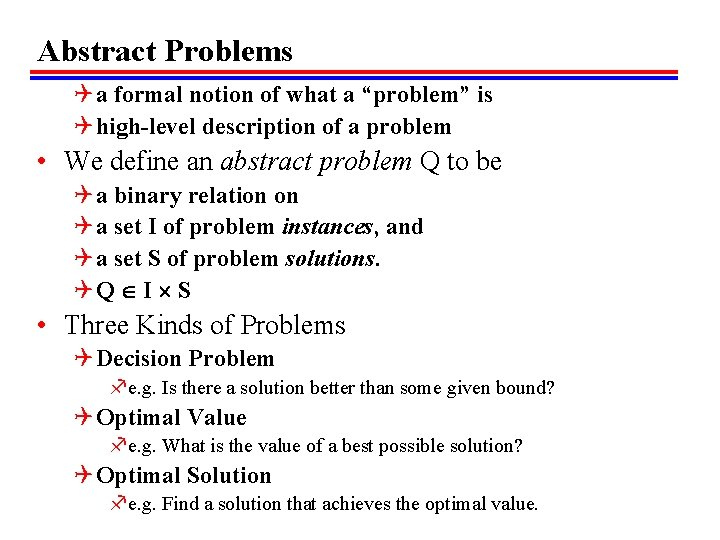Abstract Problems Q a formal notion of what a “problem” is Q high-level description of a problem • We define an abstract problem Q to be Q a binary relation on Q a set I of problem instances, and Q a set S of problem solutions. QQ I S • Three Kinds of Problems Q Decision Problem fe. g. Is there a solution better than some given bound? Q Optimal Value fe. g. What is the value of a best possible solution? Q Optimal Solution fe. g. Find a solution that achieves the optimal value.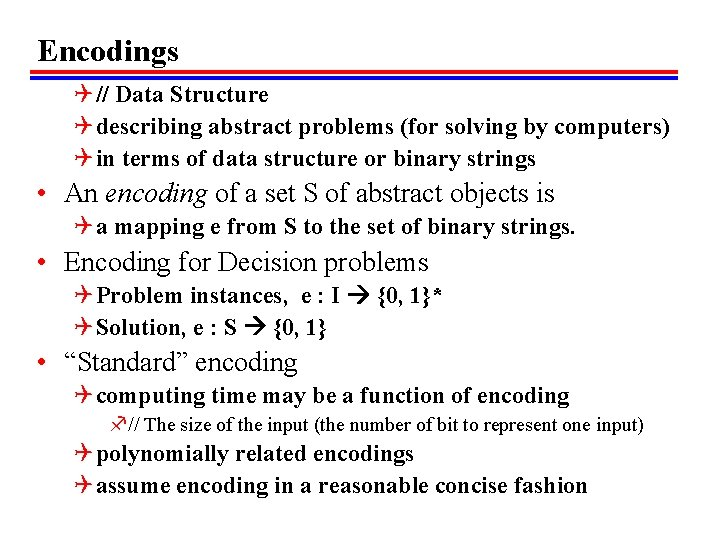Encodings Q // Data Structure Q describing abstract problems (for solving by computers) Q in terms of data structure or binary strings • An encoding of a set S of abstract objects is Q a mapping e from S to the set of binary strings. • Encoding for Decision problems Q Problem instances, e : I {0, 1}* Q Solution, e : S {0, 1} • “Standard” encoding Q computing time may be a function of encoding f// The size of the input (the number of bit to represent one input) Q polynomially related encodings Q assume encoding in a reasonable concise fashion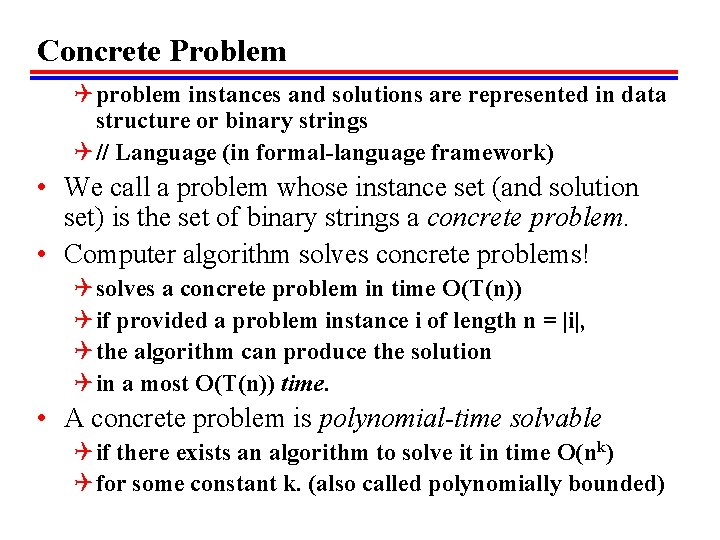Concrete Problem Q problem instances and solutions are represented in data structure or binary strings Q // Language (in formal-language framework) • We call a problem whose instance set (and solution set) is the set of binary strings a concrete problem. • Computer algorithm solves concrete problems! Q solves a concrete problem in time O(T(n)) Q if provided a problem instance i of length n = |i|, Q the algorithm can produce the solution Q in a most O(T(n)) time. • A concrete problem is polynomial-time solvable Q if there exists an algorithm to solve it in time O(nk) Q for some constant k. (also called polynomially bounded)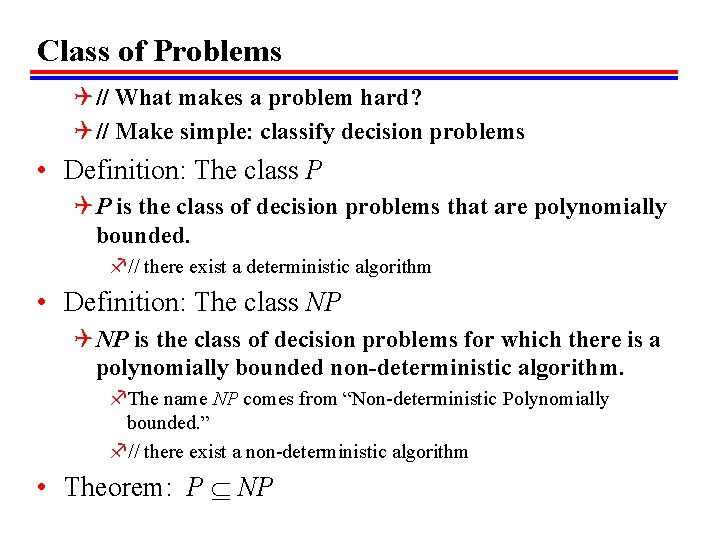Class of Problems Q // What makes a problem hard? Q // Make simple: classify decision problems • Definition: The class P Q P is the class of decision problems that are polynomially bounded. f// there exist a deterministic algorithm • Definition: The class NP Q NP is the class of decision problems for which there is a polynomially bounded non-deterministic algorithm. f. The name NP comes from “Non-deterministic Polynomially bounded. ” f// there exist a non-deterministic algorithm • Theorem: P NP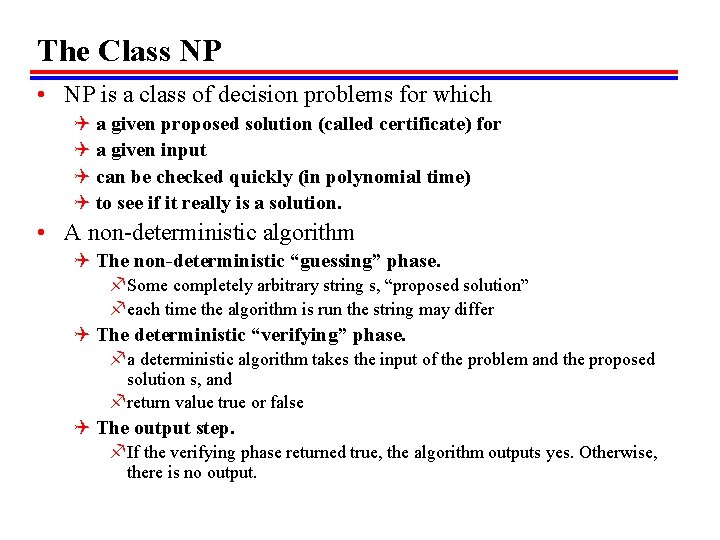The Class NP • NP is a class of decision problems for which Q a given proposed solution (called certificate) for Q a given input Q can be checked quickly (in polynomial time) Q to see if it really is a solution. • A non-deterministic algorithm Q The non-deterministic “guessing” phase. f Some completely arbitrary string s, “proposed solution” f each time the algorithm is run the string may differ Q The deterministic “verifying” phase. f a deterministic algorithm takes the input of the problem and the proposed solution s, and f return value true or false Q The output step. f If the verifying phase returned true, the algorithm outputs yes. Otherwise, there is no output.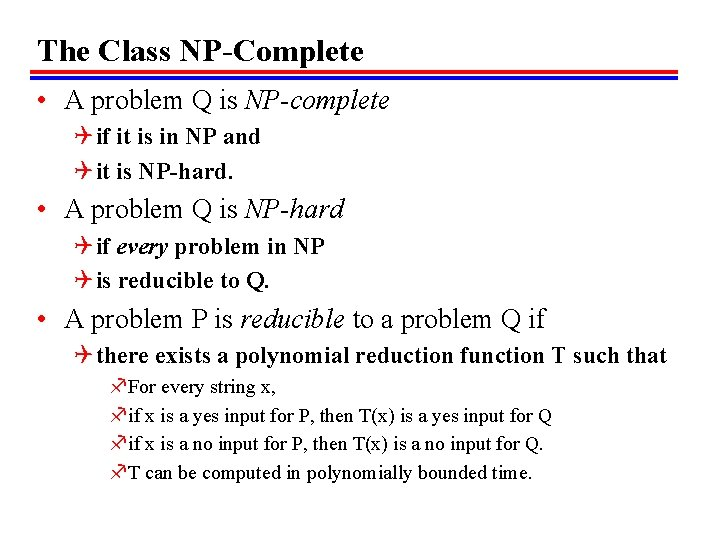The Class NP-Complete • A problem Q is NP-complete Q if it is in NP and Q it is NP-hard. • A problem Q is NP-hard Q if every problem in NP Q is reducible to Q. • A problem P is reducible to a problem Q if Q there exists a polynomial reduction function T such that f. For every string x, fif x is a yes input for P, then T(x) is a yes input for Q fif x is a no input for P, then T(x) is a no input for Q. f. T can be computed in polynomially bounded time.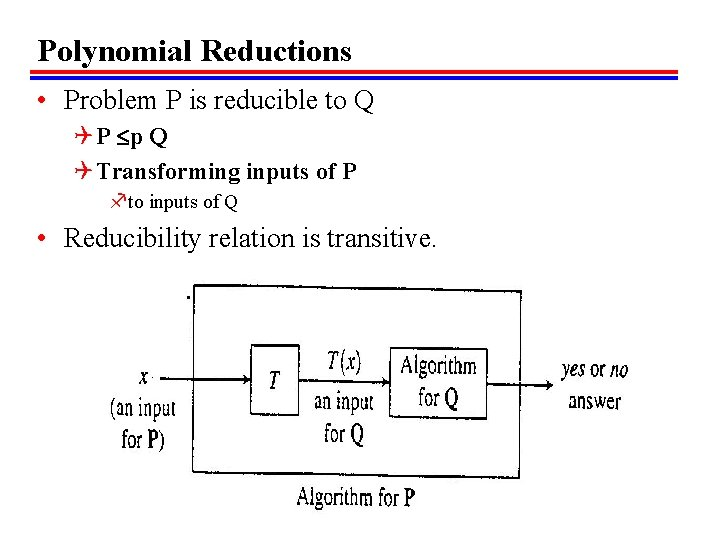Polynomial Reductions • Problem P is reducible to Q Q P p Q Q Transforming inputs of P fto inputs of Q • Reducibility relation is transitive.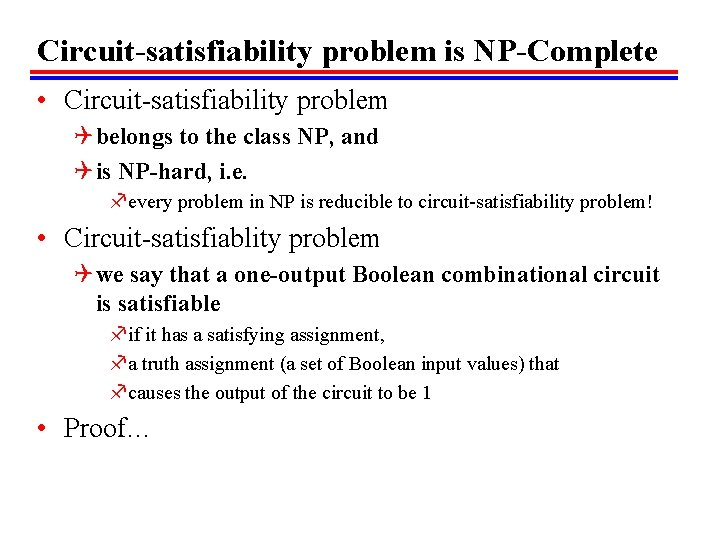Circuit-satisfiability problem is NP-Complete • Circuit-satisfiability problem Q belongs to the class NP, and Q is NP-hard, i. e. fevery problem in NP is reducible to circuit-satisfiability problem! • Circuit-satisfiablity problem Q we say that a one-output Boolean combinational circuit is satisfiable fif it has a satisfying assignment, fa truth assignment (a set of Boolean input values) that fcauses the output of the circuit to be 1 • Proof…NP-Completeness Proofs Q Once we proved a NP-complete problem • To show that the problem Q is NP-complete, Q choose a know NP-complete problem P Q reduce P to Q • The logic is as follows: Q since P is NP-complete, fall problems R in NP are reducible to P, R p P. Q show P p Q Q then all problem R in NP satisfy R p Q, fby transitivity of reductions Q therefore Q is NP-completeSolving hard problems: Approximation Algorithms Q an algorithm that returns near-optimal solutions Q may use heuristic methods fe. g. greedy heuristics • Definition: Approximation algorithm Q An approximation algorithm for a problem is Q a polynomial-time algorithm that, Q when given input I, outputs an element of FS(I). • Definition: Feasible solution set Q A feasible solution is Q an object of the right type but not necessarily an optimal one. Q FS(I) is the set of feasible solutions for I.Approximation Algorithm e. g. Bin Packing Q How to pack or store objects of various sizes and shapes Q with a minimum of wasted space • Bin Packing Q Let S = (s 1, …, sn) f where 0 < si <= 1 for 1 <= i <= n Q pack s 1, …, sn into as few bin as possible fwhere each bin has capacity one • Optimal solution for Bin Packing Q considering all ways to Q partition S into n or fewer subsets Q there are more than Q (n/2)n/2 possible partitions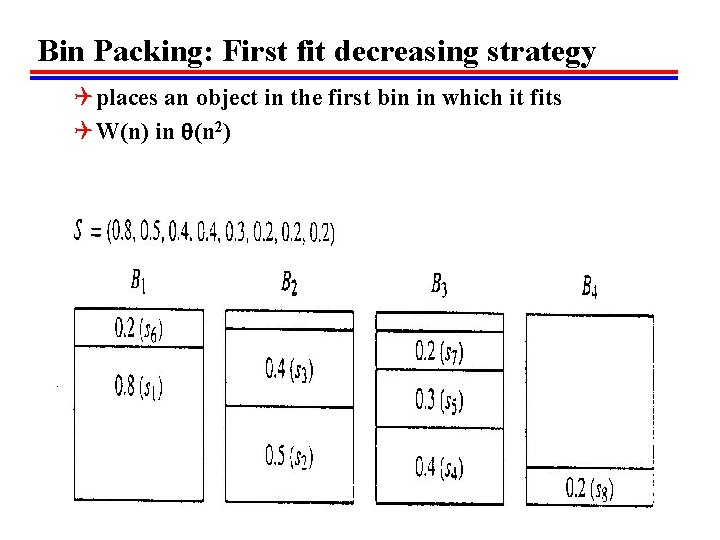Bin Packing: First fit decreasing strategy Q places an object in the first bin in which it fits Q W(n) in (n 2)Algorithm: Bin Packing (first fit decreasing) Q Input: A sequence S=(s 1, …. , sn) of type float, where 0<si<1 for 1<=i<=n. S represents the sizes of objects {1, . . . , n} to be placed in bins of capacity 1. 0 each. Q Output: An array bin where for 1<=i<=n, bin[i] is the number of the bin into which object i is placed. For simplicity, objects are indexed after being sorted in the algorithm. The array is passed in and the algorithm fills it. • • • • binpack. FFd(S, n, bin) float[] used=new float[n+1]; //used[j] is the amount of space in bin j already used up. int i, j; Initialize all used entries to 0. 0 Sort S into descending(nonincreasing)order, giving the sequence s 1>=S 2>=…>=Sn. for(i=1; i<=n; i++) //Look for a bin in which s[i] fits. for(j=1; j<=n; j++) if(used[j]+si<+1. 0) bin[i]=j; used[j] += si; break; //exit for(j) //continue for(i).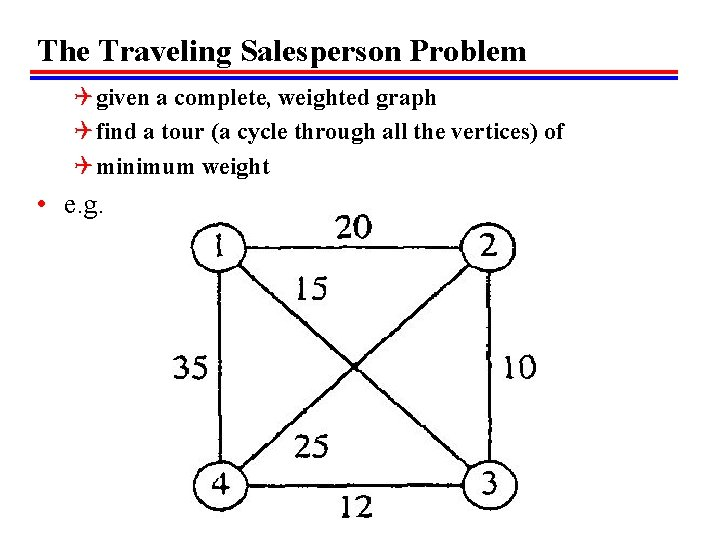The Traveling Salesperson Problem Q given a complete, weighted graph Q find a tour (a cycle through all the vertices) of Q minimum weight • e. g.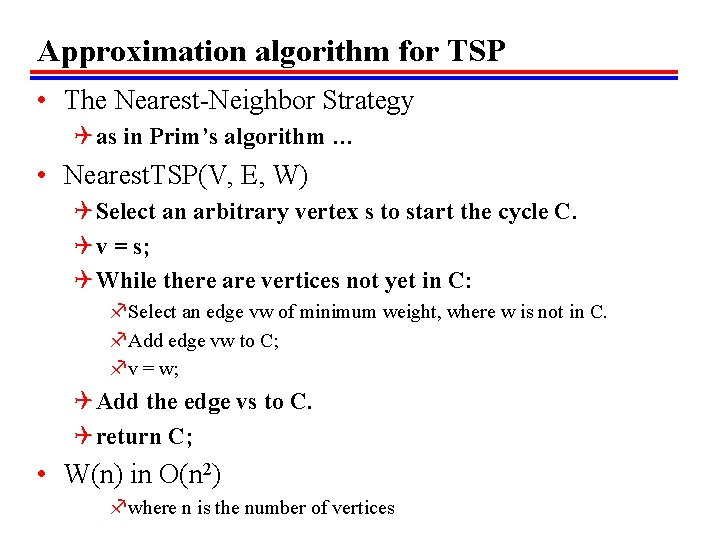Approximation algorithm for TSP • The Nearest-Neighbor Strategy Q as in Prim’s algorithm … • Nearest. TSP(V, E, W) Q Select an arbitrary vertex s to start the cycle C. Q v = s; Q While there are vertices not yet in C: f. Select an edge vw of minimum weight, where w is not in C. f. Add edge vw to C; fv = w; Q Add the edge vs to C. Q return C; • W(n) in O(n 2) fwhere n is the number of vertices